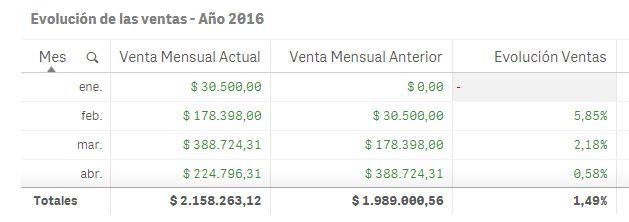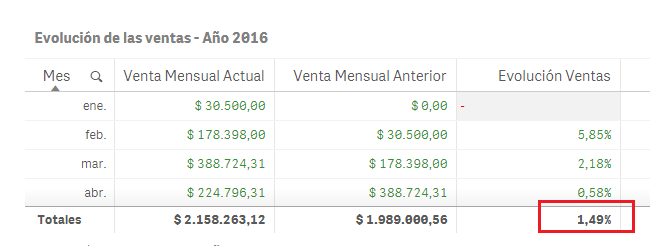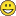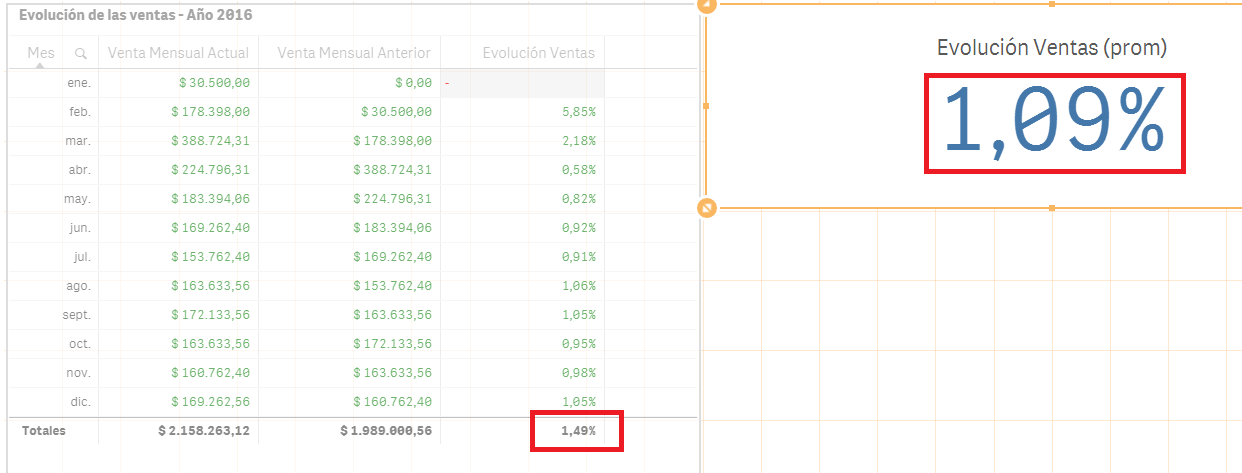# App Development

Announcements
Skip the ticket, Chat with Qlik Support instead for instant assistance.
cancel
Showing results for
Did you mean:Anonymous
Not applicable

## Totals in a table as a KPI

Hi everybody,

I've beeing reading lot of posts to find an answer to my doubt but I can´t reach the right one, so I decided to make a post.

I have this table:Where:

Venta Mensual actual = Sum(IF(IDCuentaContable=40, Monto))

Venta mensual Anterior = Sum(IF(IDCuentaContable=40, MontoAnterior))

Evolucion ventas = Sum(IF(IDCuentaContable=40, Monto))/Sum(IF(IDCuentaContable=40, MontoAnterior))/100

All of them are master elements.

I need to filter only account No 40.

Monto Anterior was created from script as follow:

IF(((Mes-1)=0),0, (Previous(Monto))) as MontoAnterior

I want to show the Total of Evolucion Ventas (1.49%) as a KPI

I've tried with a lot of functions but I can´t do it.

Could I have some help? I´m making my first steps with Qlik Sense

1 Solution

Accepted SolutionsMVP

Try this

Avg(Aggr(Sum({<IDCuentaContable={40}>}Monto)/Sum({<IDCuentaContable={40}>}MontoAnterior)/100, Mes))

9 RepliesMVP

What is the total mode for Evolucion Ventas measure?Anonymous
Not applicable
Author

Hi Sunny,

I´m not sure what your question is.

I need to show the red mark as a KPII put the script in my previous postMVP

I am not sure how you are calculating 1.49%... can you explain how you get this number?Contributor III

i'm  pretty new myself but since you just need the totals as a kpi couldn't you just put your function:

Sum(IF(IDCuentaContable=40, Monto))/Sum(IF(IDCuentaContable=40, MontoAnterior))/100

into a kpi and get the right result. since the only real difference in the table is that it is grouped by a dimension months.

i would also stongly recommend you don't use The IF function inside a sum of any other  aggragation function as this severely slows down your front end application. so your set analysis should look like this:

sum({<IDCuentaContable = {40}>}, Monto)/ sum({<IDCuentaContable=40>}, MontoAnterior)/100

Elsewise if this doesn't work i think you can do something like this

sum(aggr(months, sum({<IDCuentaContable = {40}>}, Monto)/ sum({<IDCuentaContable=40>}, MontoAnterior)/100))

this wil first create a table with all evolucion ventas per month and then just make the sum out of it.

Could be i'm misinterpreting your question so pleas elaborate if this is wrongAnonymous
Not applicable
Author

Hi Sunny, let me explain you a bit more.

This is the complete table:"Totales" is automatically calculated by Qlik

I made the same process in excel and the values are right

I need to reproduce them in KPIsAnonymous
Not applicable
Author

Hi Jelle,

thanks for you advice with IF function!

I tried the options you gave me and these was the results:

1) The script Sum({<IDCuentaContable={40}>}Monto)/Sum({<IDCuentaContable={40}>}MontoAnterior)/100 results in differences between the KPI and the column "Evolucion ventas" as show:And I noticed this:

if you do 2.158.263,13 / 1.989.000.56 the result is 1.09%

But, if you calculate the average of Evolucion Ventas column, the result will be 1.49%

So I guess that I need to make an script to calculate the average of the last column (take in account that Totales are automatically calculated by Qlik). And if you repete these calculus in excel they are correct

2) The second script didn´t work:

Sum(aggr(Mes, (sum({<IDCuentaContable = {40}>} Monto)/ sum({<IDCuentaContable={40}>} MontoAnterior)/100)))MVP

Try this

Avg(Aggr(Sum({<IDCuentaContable={40}>}Monto)/Sum({<IDCuentaContable={40}>}MontoAnterior)/100, Mes))MVP

Did you give this a shot?

Avg(Aggr(Sum({<IDCuentaContable={40}>}Monto)/Sum({<IDCuentaContable={40}>}MontoAnterior)/100, Mes))Anonymous
Not applicable
Author

Thank you Sunny, your script worked!!

I really appreciate you help!Tags
Community Browser